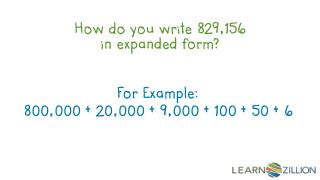DownloadDownload PresentationHow do you write 829,156 in expanded form?

# How do you write 829,156 in expanded form?

Download Presentation## How do you write 829,156 in expanded form?

- - - - - - - - - - - - - - - - - - - - - - - - - - - E N D - - - - - - - - - - - - - - - - - - - - - - - - - - -
##### Presentation Transcript

1. How do you write 829,156 in expanded form? For Example: 800,000 + 20,000 + 9,000 + 100 + 50 + 6

2. In this lesson, you will learn how to read and write numbers in expanded form by using arrow cards.

3. thousands hundreds ones tens 4 0 0 0 5 0 0 3 0 2 3 0 2 5 0 0 4 0 0 0

4. Thinking that the number 2 in 32,586 has a value of 2 instead of 2,000.

5. What is expanded form? 7 0 0 0 8 0 0 2 0 6 0 0 0 0 1 1 6 0 0 0 0 + 2 0 + + 7 0 0 0 8 0 0 +

6. Expanded Form 6 7 , 8 2 1 1 20 + 800 + 7,000 + 60,000 +

7. 600,000 + 50,000 + 4,000 + 400 + 30 + 9 600,000 50,000 4,000 400 30 + 9 6 4 5 4 3 9 , - - - - - - 654,439

8. 1000 500 40 + 5 40 + 1000 + 5 + 500 1000 + 500 + 40 + 5 1,545 1,545

9. 4 tens + 50 tens + 1 thousand + 5 ones 40 500 5 1,000 1000 500 40 + 5 1000 + 500 + 40 + 5 1,545 1,545

10. In this lesson, you have learned learn how to read and write numbers in expanded form by using arrow cards.

11. Write 829,156 in expanded form.

12. Use arrow cards to create the following numbers. In your math journal, rewrite these numbers in expanded form. • 58,352 • 7,399,815 • 3,459,242

13. Roll a dice 7 times to create a random number. Write each digit on a place value chart like the one shown below. • Write this number in numeric, word, and expanded form.

14. 1) What is 56,399 in expanded form?

15. 2) How would you write: 3,000 + 40,000 + 2 + 50 + 200 + 900,000 in numeric form?